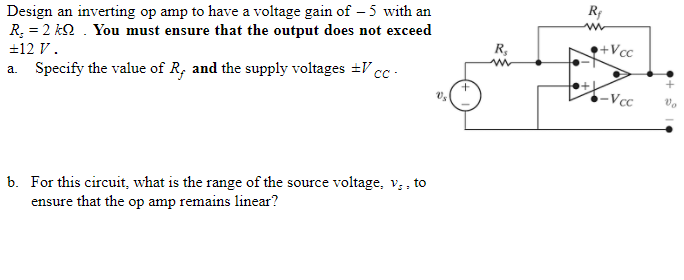# Question R Design an inverting op amp to have a voltage gain of - 5 with an R: = 2 kYou must ensure that the output does not exceed +12 V. a. Specify the value of R, and the supply voltages =VCC R, •+Vcc 0. -VCC b. For this circuit, what is the range of the source voltage, V., to ensure that the op amp remains linear?JZOLYH The Asker · Electrical EngineeringTranscribed Image Text: R Design an inverting op amp to have a voltage gain of - 5 with an R: = 2 kYou must ensure that the output does not exceed +12 V. a. Specify the value of R, and the supply voltages =VCC R, •+Vcc 0. -VCC b. For this circuit, what is the range of the source voltage, V., to ensure that the op amp remains linear?
More
Transcribed Image Text: R Design an inverting op amp to have a voltage gain of - 5 with an R: = 2 kYou must ensure that the output does not exceed +12 V. a. Specify the value of R, and the supply voltages =VCC R, •+Vcc 0. -VCC b. For this circuit, what is the range of the source voltage, V., to ensure that the op amp remains linear?